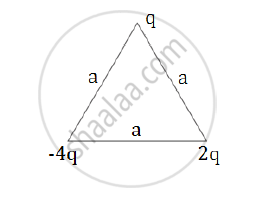# Obtain an Expression for the Work Done to Dissociate the System of Three Charges - Physics

Obtain an expression for the work done to dissociate the system of three charges placed at the vertices of an equilateral triangle of side ‘a’ as shown below.#### Solution

Work done to keep the system bound is

W=(Kq_1q_2)/a+(Kq_2q_3)/a+(Kq_1q_3)/a

W=(Kq(-4q))/a+(K(-4q)2q)/a+(Kq(2q))/a

W=(-K4q^2)/a-(K8q^2)/a+(K2q^2)/a

W=(Kq^2)/a(-4-8+2)=(-10Kq^2)/a

Therefore, the work done to dissociate the system is

W_d=-W=(10Kq^2)/a

Concept: Forces Between Multiple Charges
Is there an error in this question or solution?